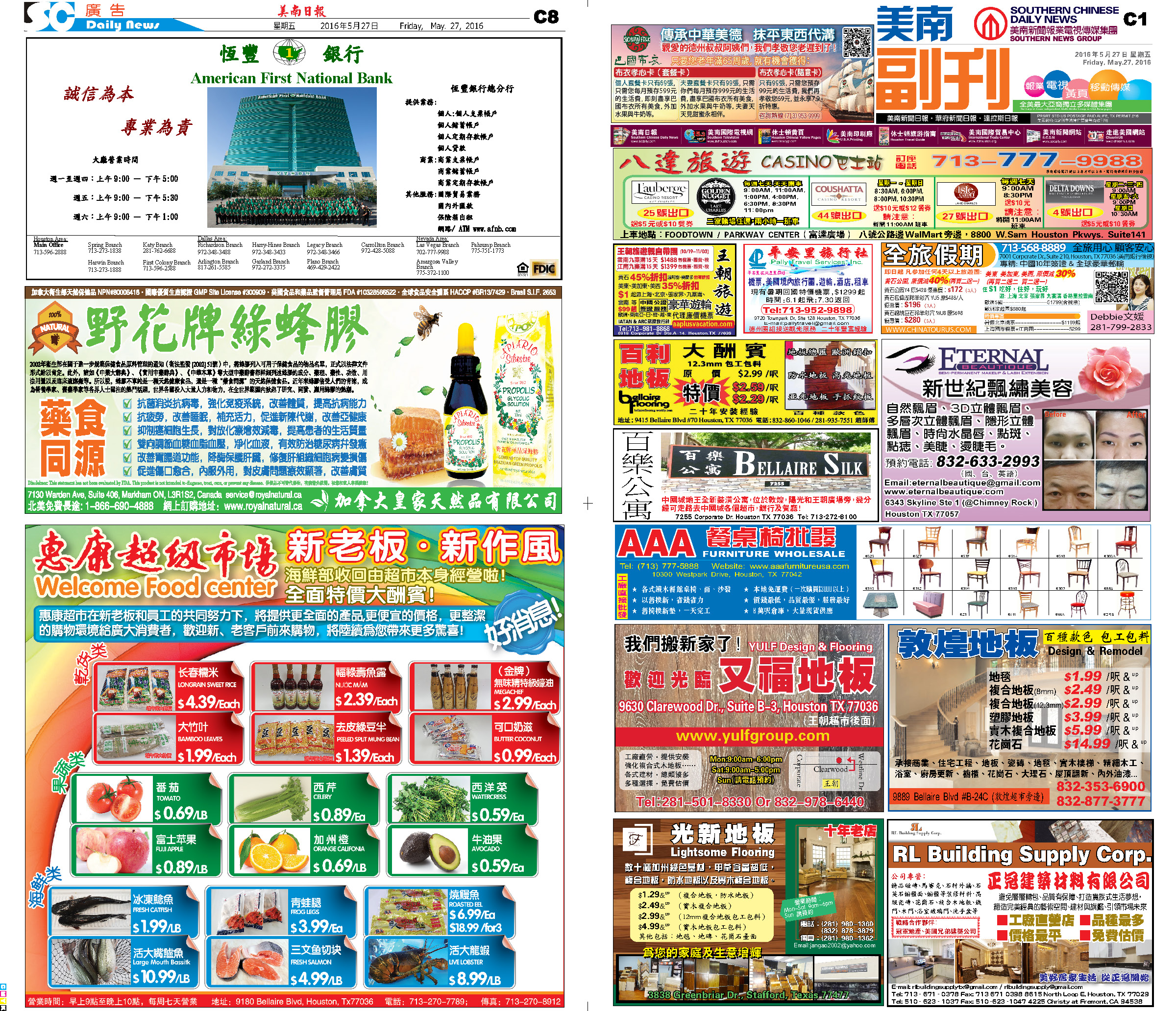160606 Epaper

 A Section B SectionC Section D Section160605 Epaper

 A Section B Section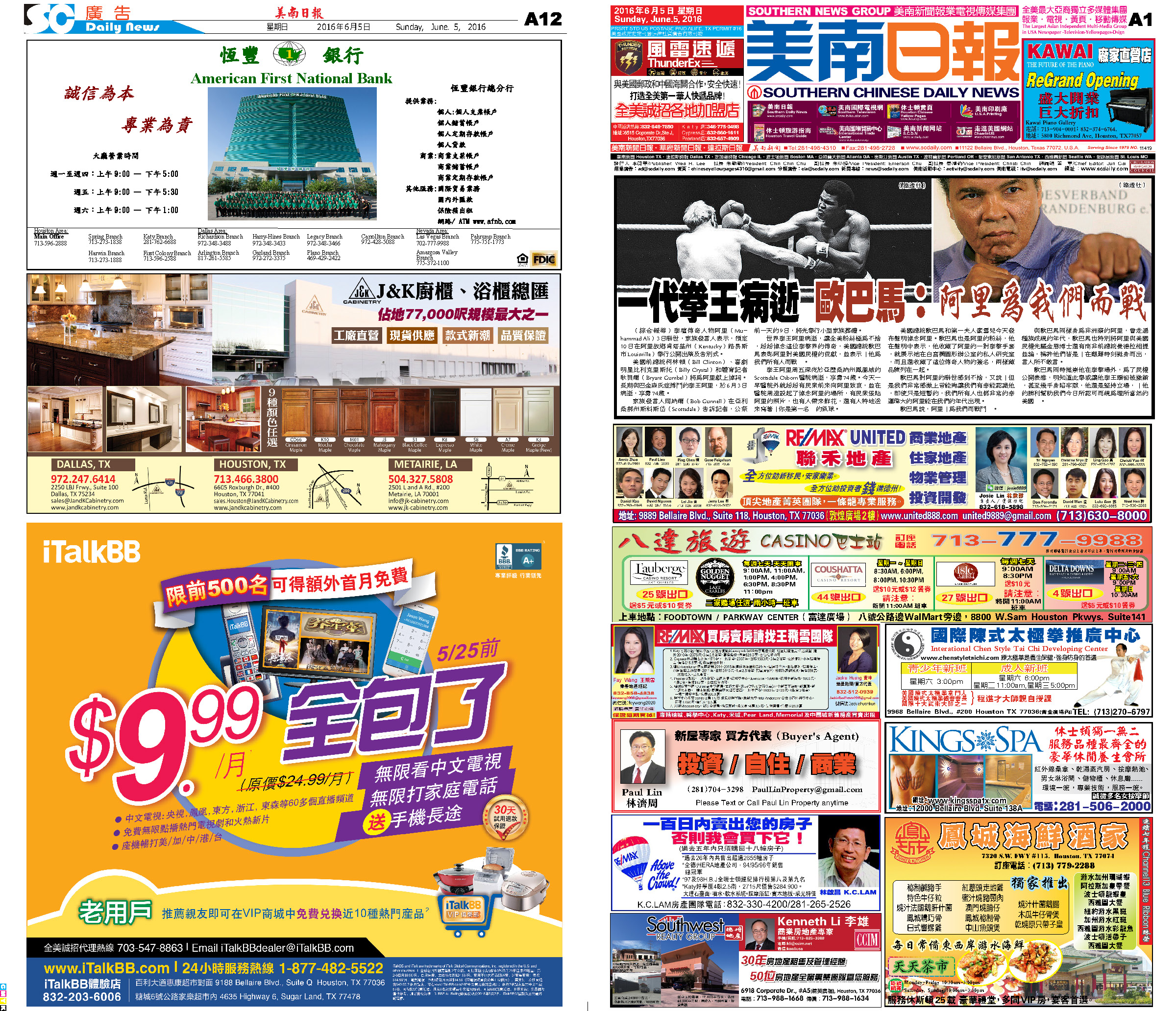C Section D Section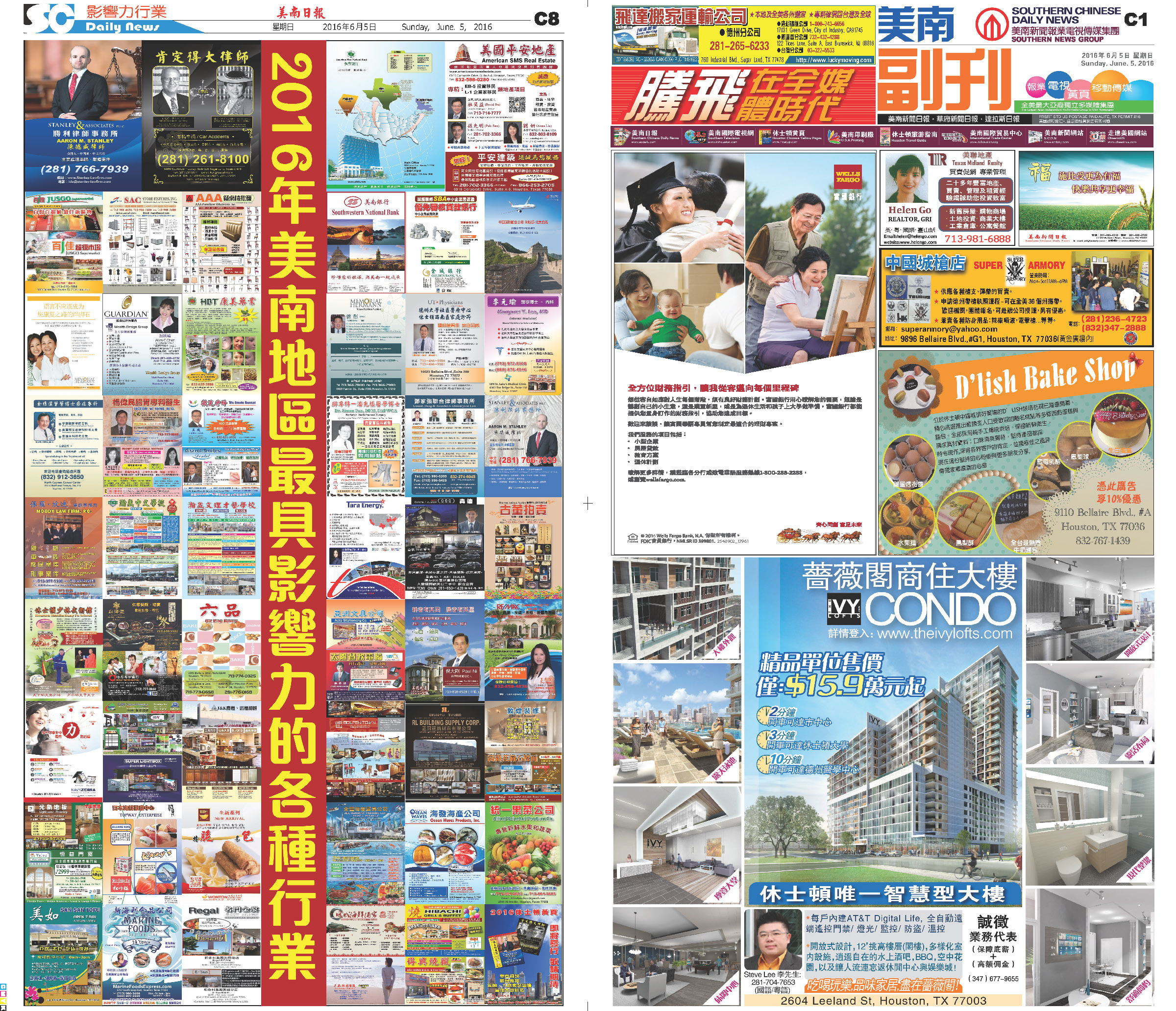160604 Epaper

 A Section B Section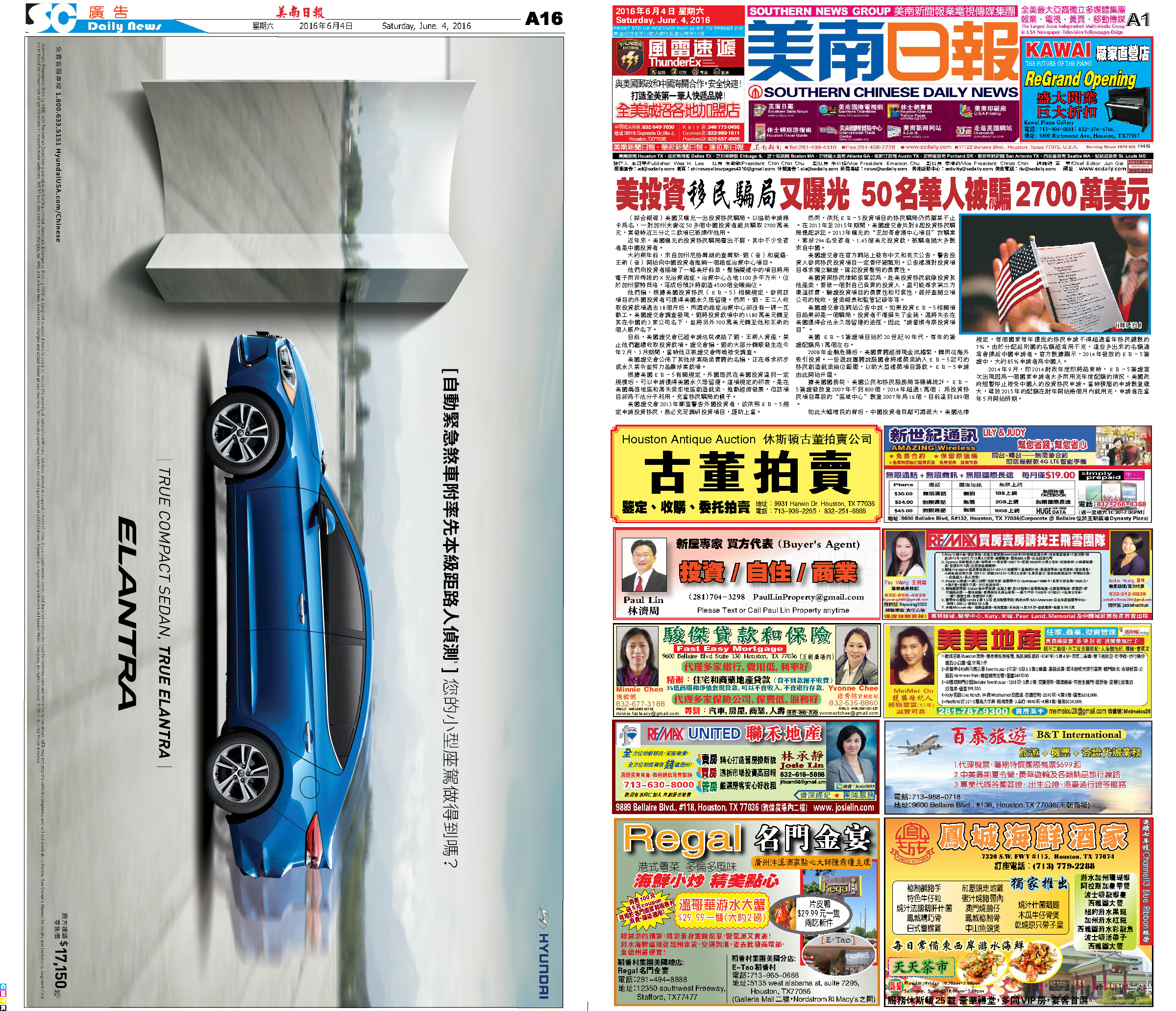C Section D Section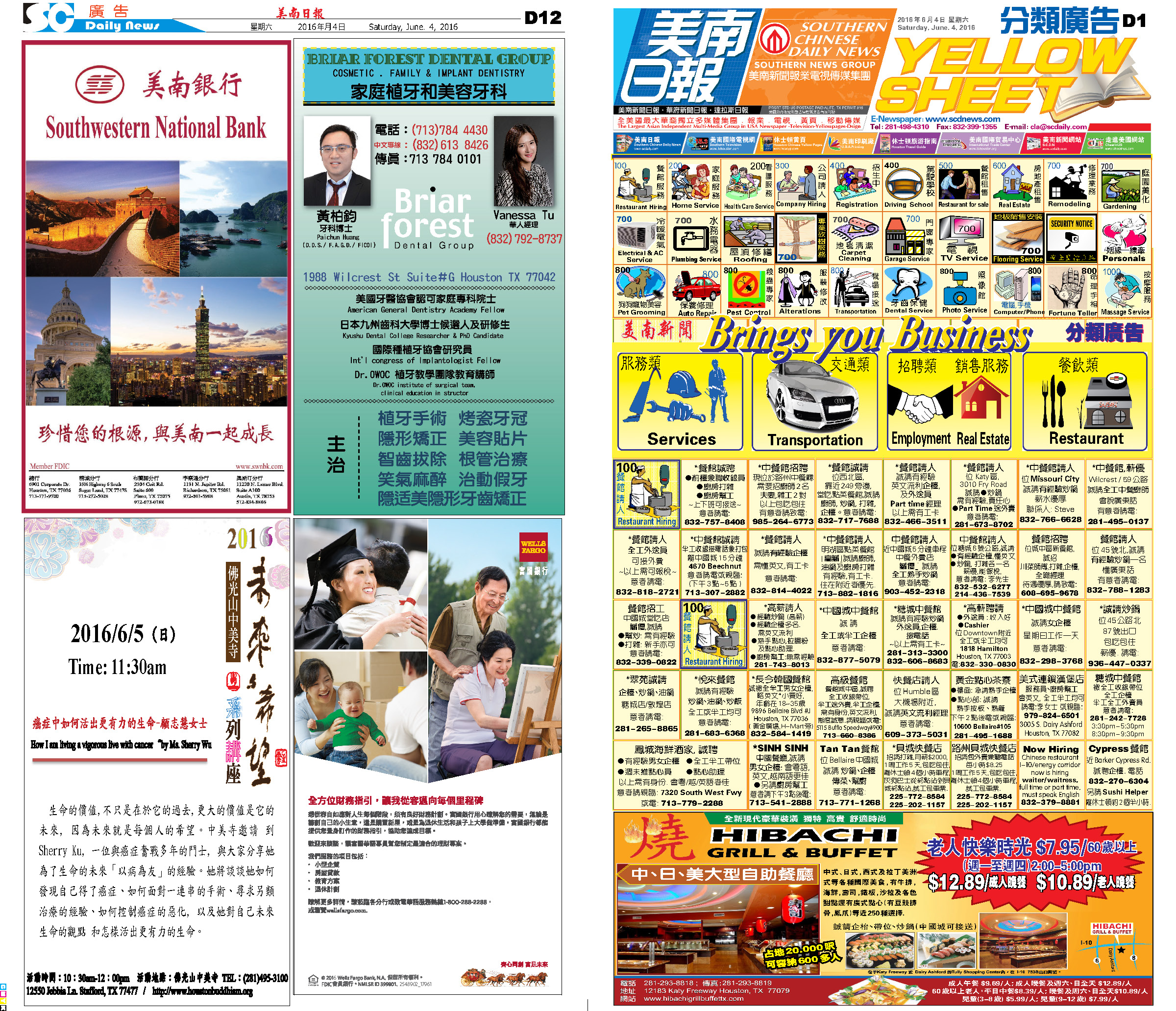160603 Epaper

 A Section B SectionC Section D Section160602 Epaper

 A Section B Section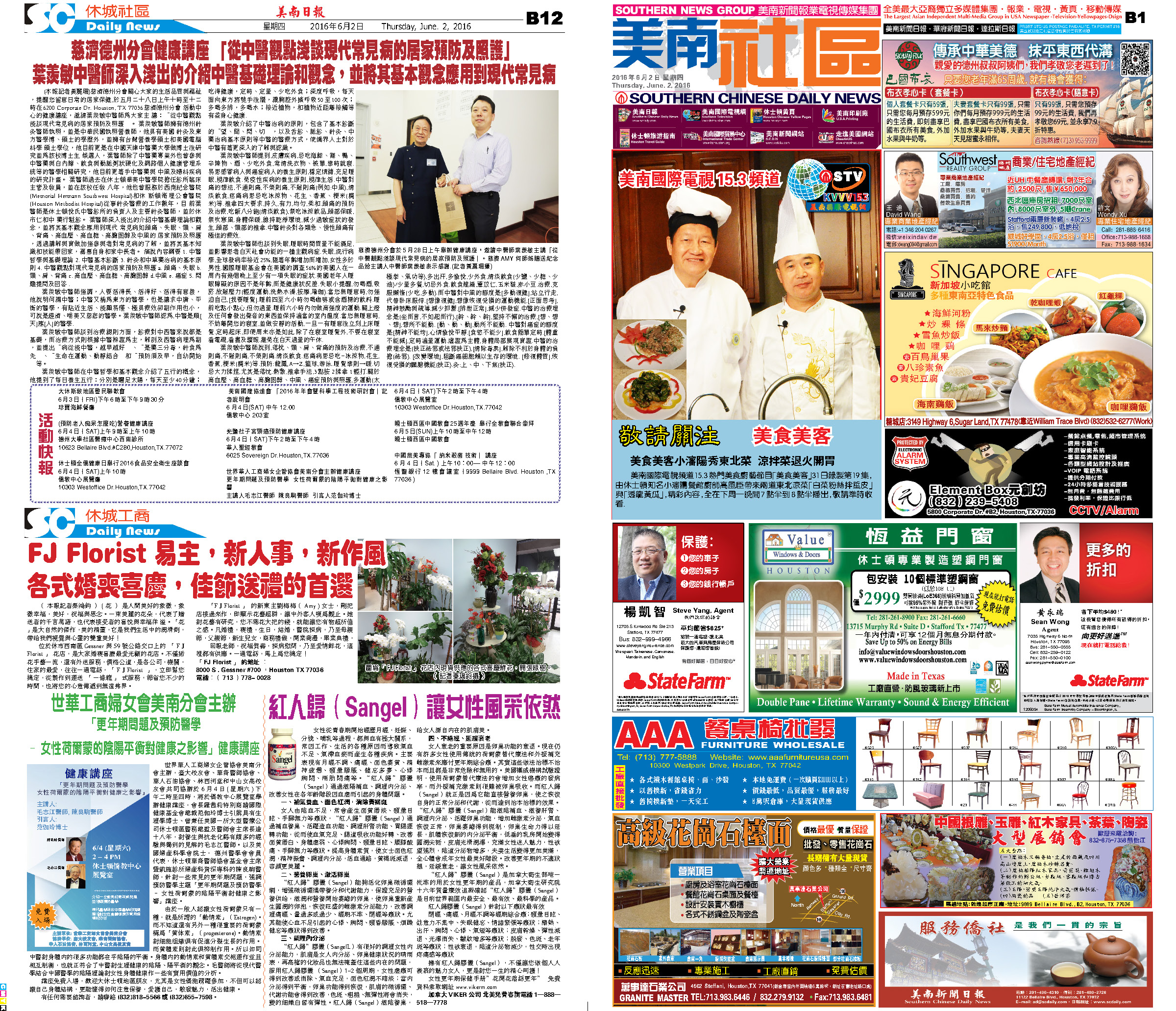C Section D Section160601 Epaper

 A Section B SectionC Section D Section160529 Epaper

 A Section B SectionC Section D Section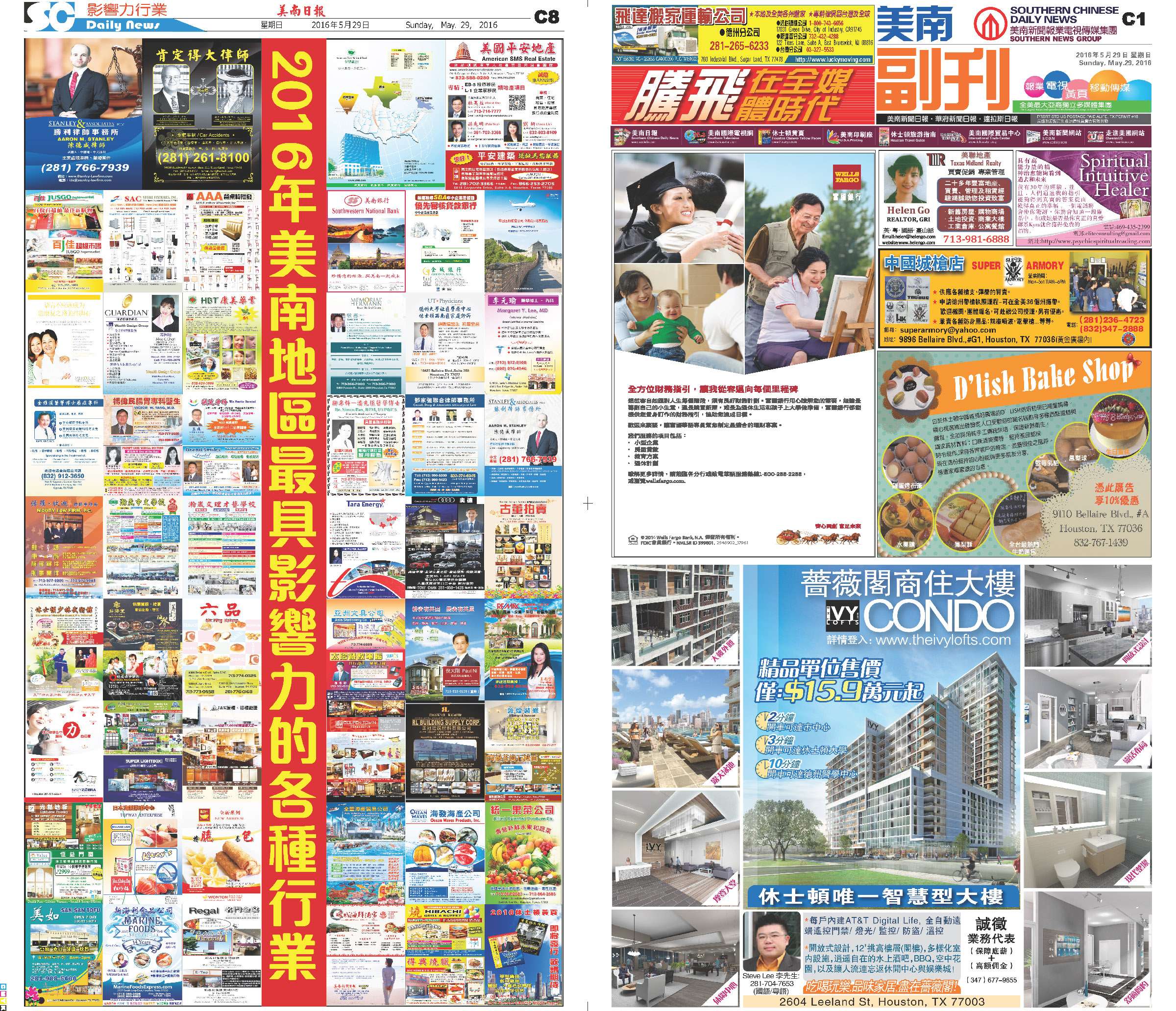160531 Epaper

 A Section B Section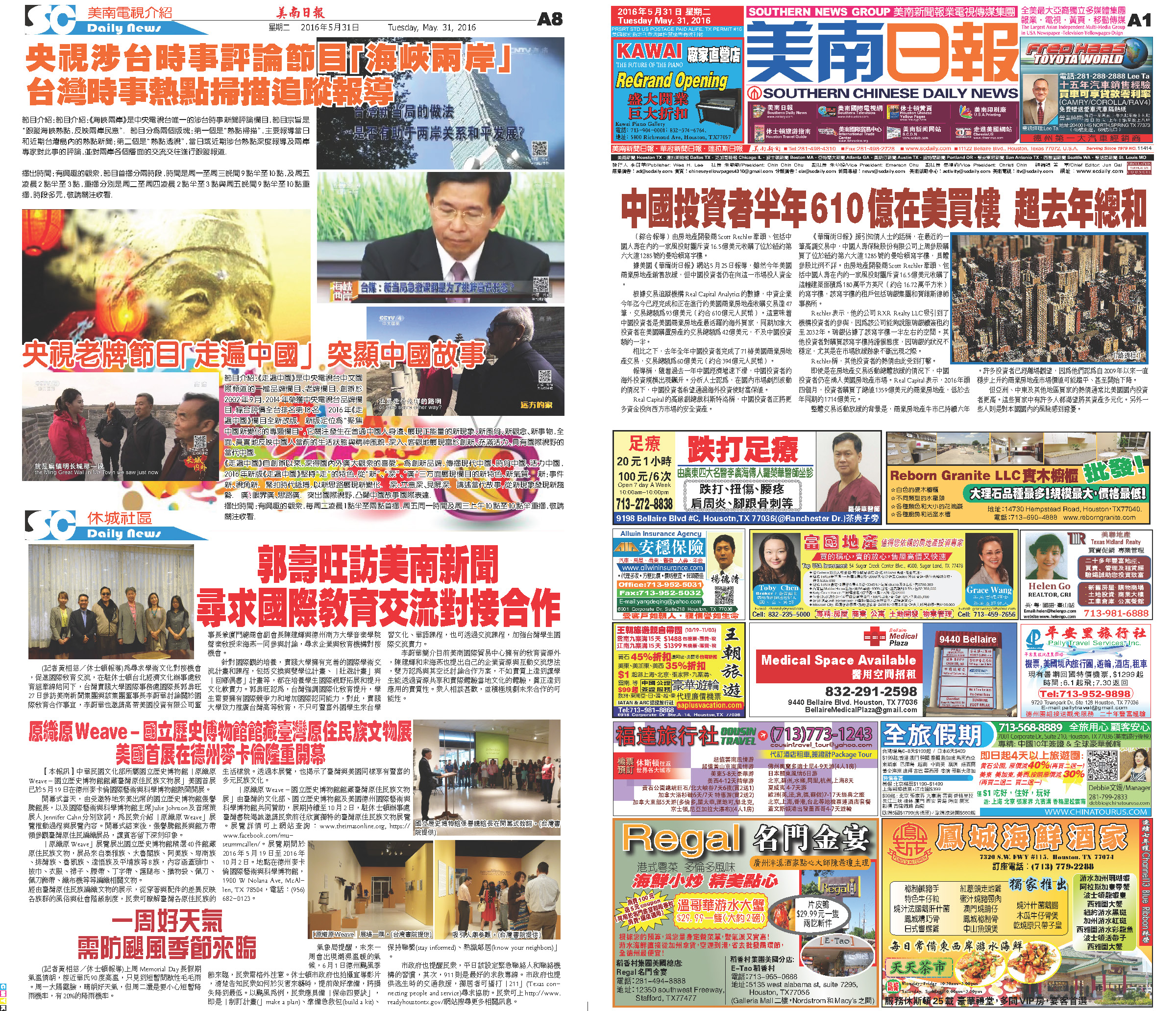C Section D Section160528 Epaper

 A Section B Section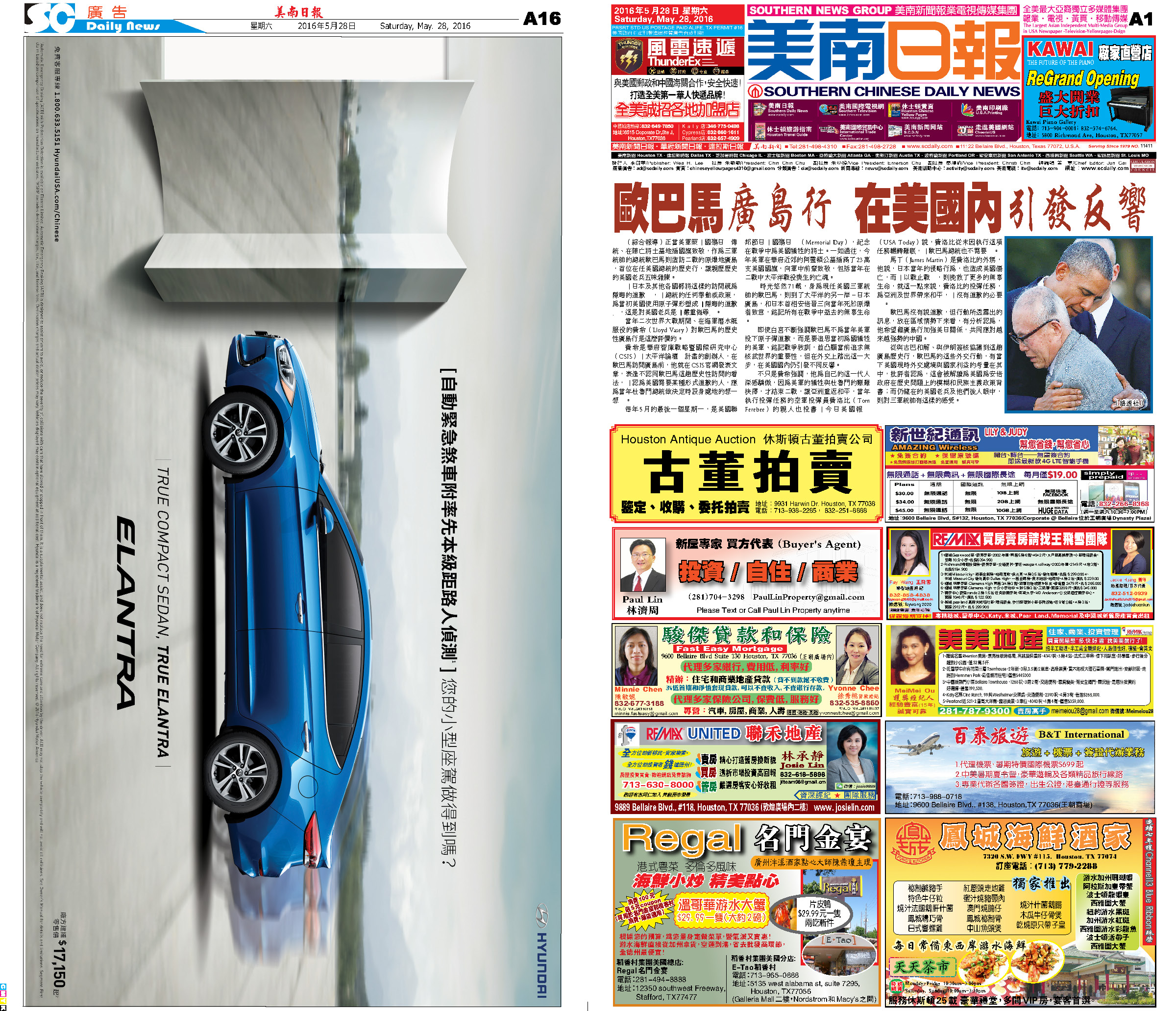C Section D Section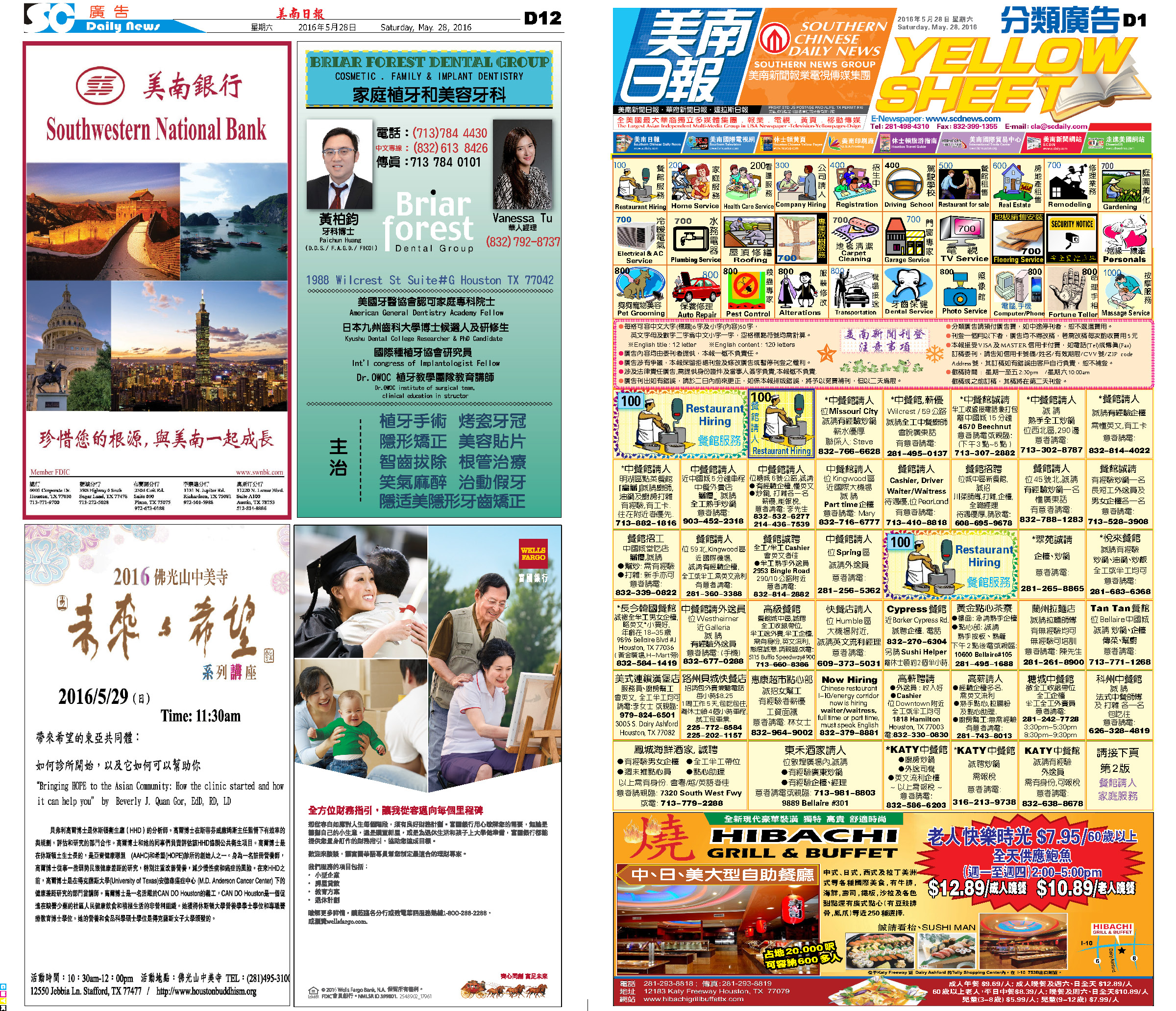160530 Epaper

 A Section B SectionC Section D Section160527 Epaper

 A Section B SectionC Section D Section